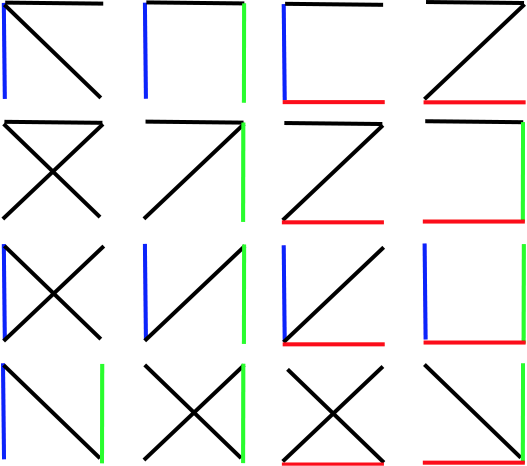February 5, 2012

No. of Spanning Trees for a Complete Graph with Constraints

For a graph with v vertices, the spanning tree contains (v-1) edges. Also the number of spanning trees can be given by vv-2 (Cayleys, 1889). Using this formula, lets determine a relationship between number of constraints and the number of spanning trees. Consider the following complete graph with 4 vertices. The colored edges will be the constraints (compulsory edges) we will consider.According to Cayleys formula there has to be 44-2=16 spanning trees for the graph and each spanning tree contains (4-1) = 3 edges. The image below shows all the spanning trees.According to the spanning trees, lets consider the number of spanning trees when constraints are present.

 # Constraints Constraints # Spanning trees 0 No constraint 16 1 Blue 8 1 Red 8 1 Green 8 2 Blue, Red 3 2 Blue, Green 4 2 Red, Green 3 3 Red, Green, Blue 1

Conclusion:

According to the above numbers, although there is a relationship when one constraint is present (Vv-2/2), there is no such linear relationship when two or more constraints are present. If the two compulsory edges are adjacent then the number of spanning tree is lesser. When the number of constraints equals the number of edges in spanning tree, only one spanning tree exists.

1 comment:

1.Wow, cool post. I'd like to write like this too - taking time and real hard work to make a great article... but I put things off too much and never seem to get started. Thanks though. 바둑이사이트